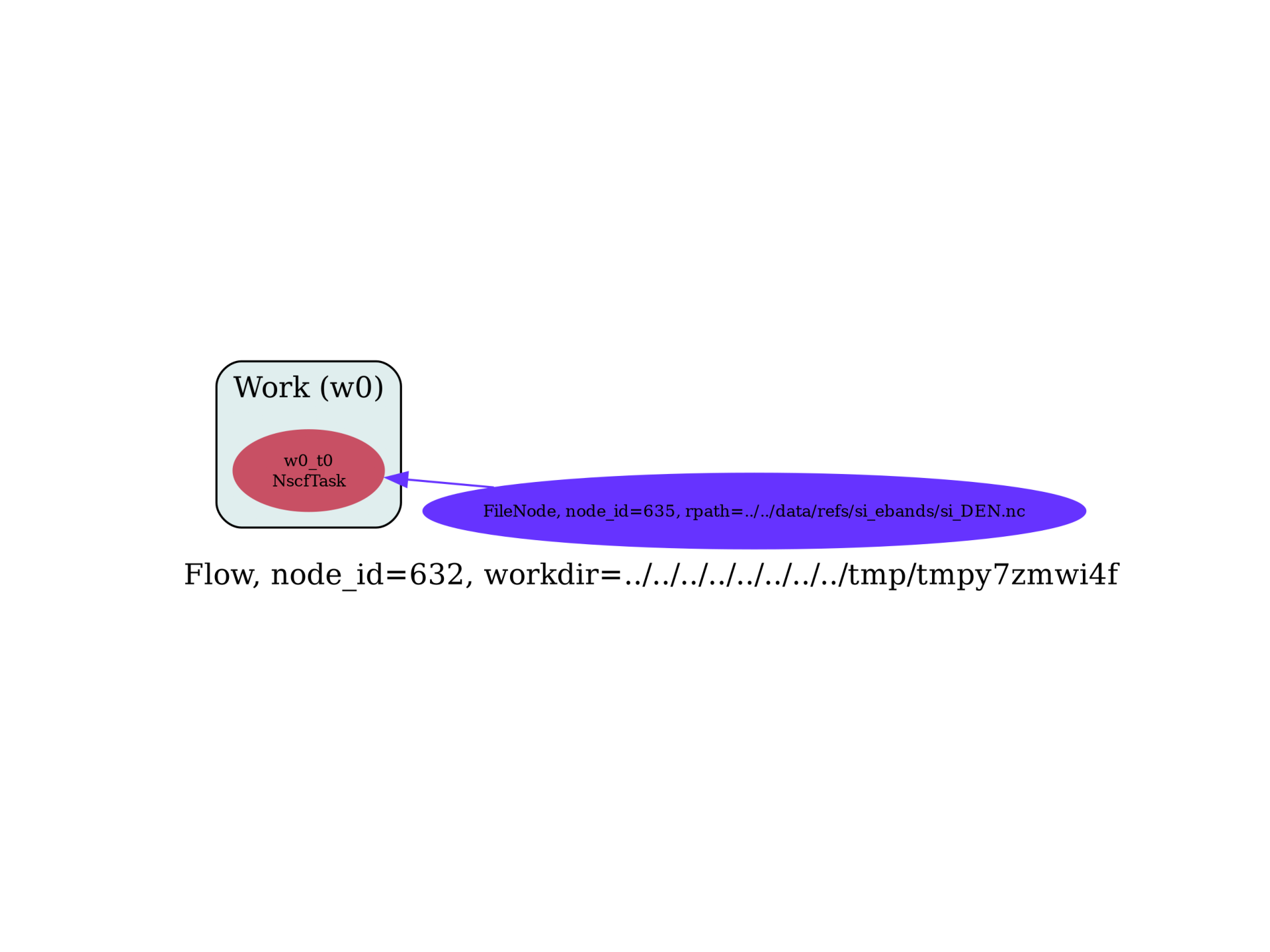# Starting a Flow from external files¶

This example shows how to build a flow for Nscf calculations in which the dependency is given by an external density file.Out:

/Users/gmatteo/git_repos/pymatgen/pymatgen/util/plotting.py:550: UserWarning: Matplotlib is currently using agg, which is a non-GUI backend, so cannot show the figure.
plt.show()


import sys
import os
import abipy.data as abidata
import abipy.abilab as abilab
import abipy.flowtk as flowtk

def make_scf_nscf_inputs(paral_kgb=1):
"""Returns two input files: GS run and NSCF on a high symmetry k-mesh."""
multi = abilab.MultiDataset(structure=abidata.cif_file("si.cif"),
pseudos=abidata.pseudos("14si.pspnc"), ndtset=2)

# Global variables
ecut = 6
global_vars = dict(
ecut=ecut,
nband=8,
nstep=15,
paral_kgb=paral_kgb,
)

if multi.ispaw:
global_vars.update(pawecutdg=2*ecut)

multi.set_vars(global_vars)

# Dataset 1 (GS run)
multi.set_kmesh(ngkpt=[8, 8, 8], shiftk=[0, 0, 0])
multi.set_vars(tolvrs=1e-6)

# Dataset 2 (NSCF run)
kptbounds = [
[0.5, 0.0, 0.0], # L point
[0.0, 0.0, 0.0], # Gamma point
[0.0, 0.5, 0.5], # X point
]

multi.set_kpath(ndivsm=6, kptbounds=kptbounds)
multi.set_vars(tolwfr=1e-12)

# Generate two input files for the GS and the NSCF run
scf_input, nscf_input = multi.split_datasets()

return scf_input, nscf_input

def build_flow(options):
# Working directory (default is the name of the script with '.py' removed and "run_" replaced by "flow_")
if not options.workdir:
options.workdir = os.path.basename(sys.argv).replace(".py", "").replace("run_", "flow_")

# Get the SCF and the NSCF input.
scf_input, nscf_input = make_scf_nscf_inputs()

# Build the flow.
flow = flowtk.Flow(options.workdir, manager=options.manager)

# Create a Work, all tasks in work will start from the DEN file.
# Note that the file must exist when the work is created
# Use the standard approach based on tasks and works if
# there's a node who needs a file produced in the future.
work = flowtk.Work()
den_filepath = abidata.ref_file("si_DEN.nc")
flow.register_work(work)

return flow

# This block generates the thumbnails in the AbiPy gallery.
# You can safely REMOVE this part if you are using this script for production runs.
__name__ = None
import tempfile
options = flowtk.build_flow_main_parser().parse_args(["-w", tempfile.mkdtemp()])
build_flow(options).graphviz_imshow()

@flowtk.flow_main
def main(options):
"""
This is our main function that will be invoked by the script.
flow_main is a decorator implementing the command line interface.
Command line args are stored in options.
"""
return build_flow(options)

if __name__ == "__main__":
sys.exit(main())


Total running time of the script: ( 0 minutes 0.475 seconds)

Gallery generated by Sphinx-Gallery